# The surprising story of the exponential function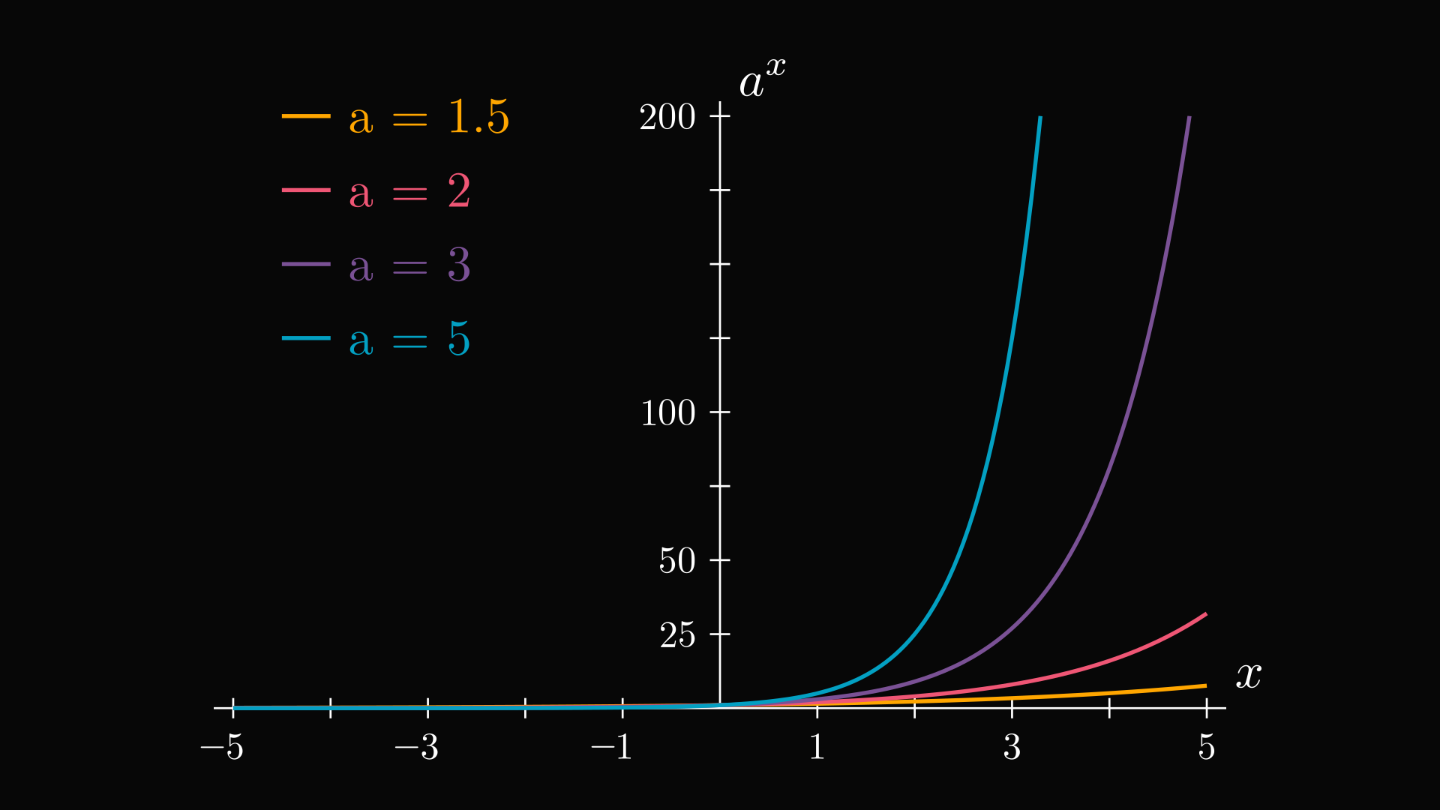The way you think about the exponential function is (probably) wrong.

Don't think so? Let me convince you. Did you realize that multiplying $e$ with itself $\pi$ times doesn't make sense?

Here is what's really behind the most important function of all time.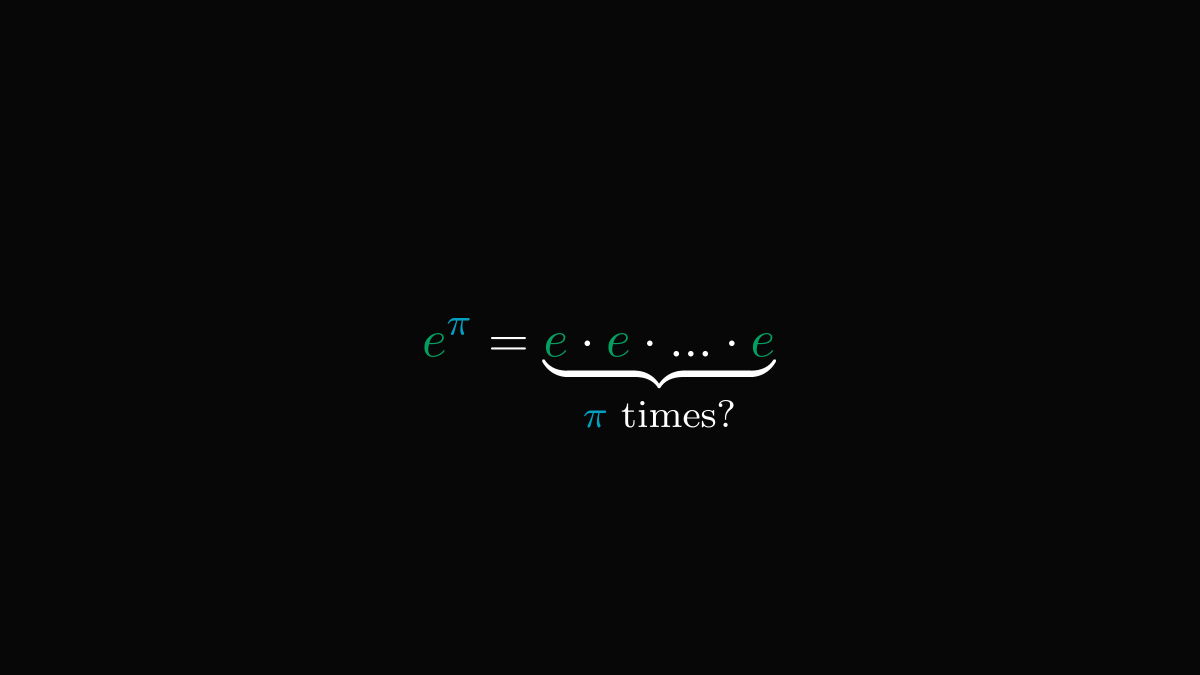First things first: terminologies. The expression $a^b$ is read "$a$ raised to the power of $b$." $a$ is called the base, and $b$ is called the exponent.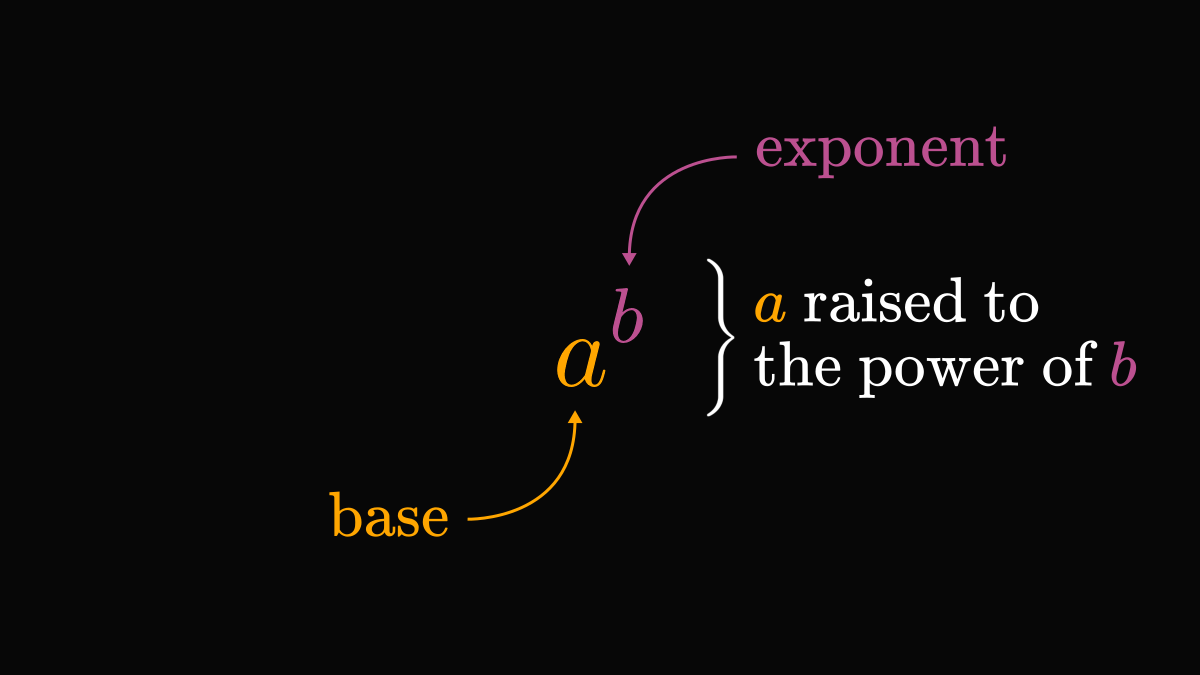Let's start with the basics: positive integer exponents. By definition, $a^n$ is the repeated multiplication of $a$ by itself $n$ times.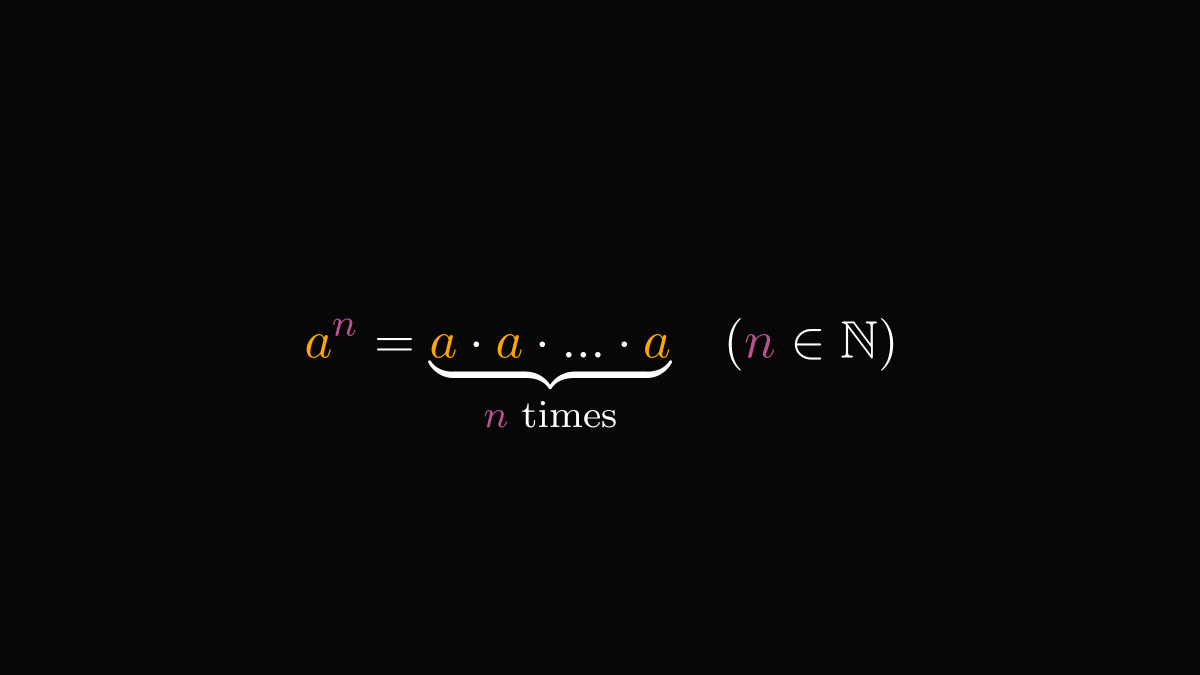Sounds simple enough. But how can we define exponentials for, say, negative integer exponents? We'll get there soon.

Two special rules will be our guiding lights. First, exponentiation turns addition into multiplication.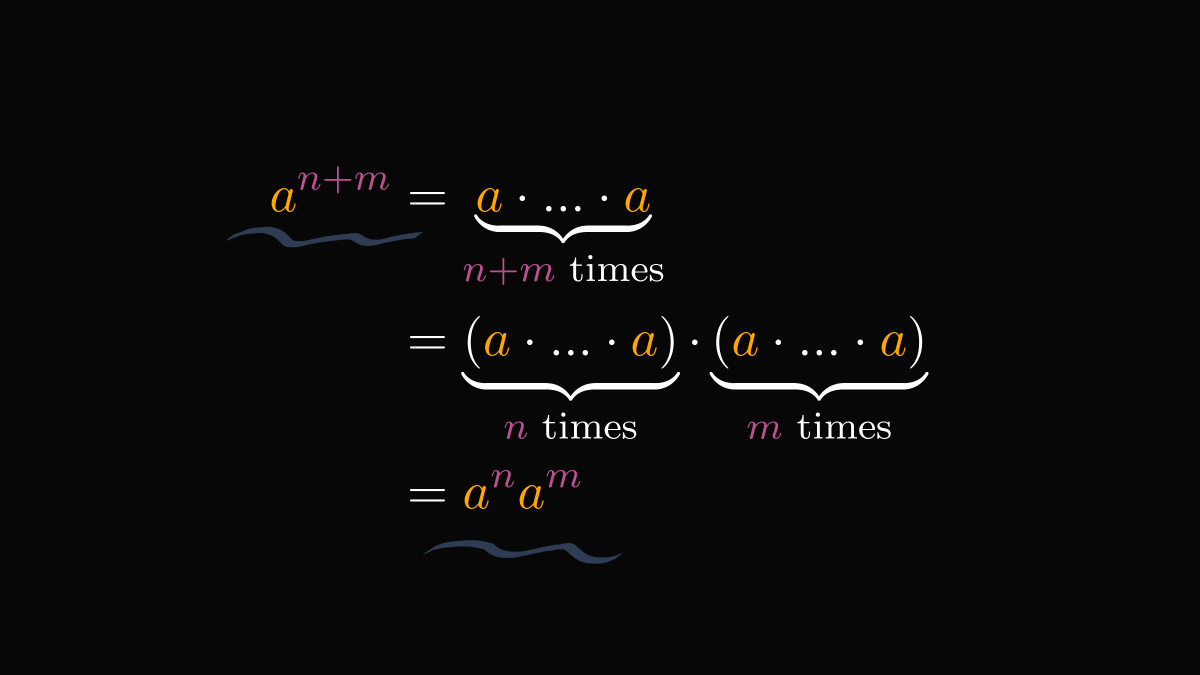We'll call this the "product of powers" rule.

Second, the repeated application of exponentiation is, again, exponentiation.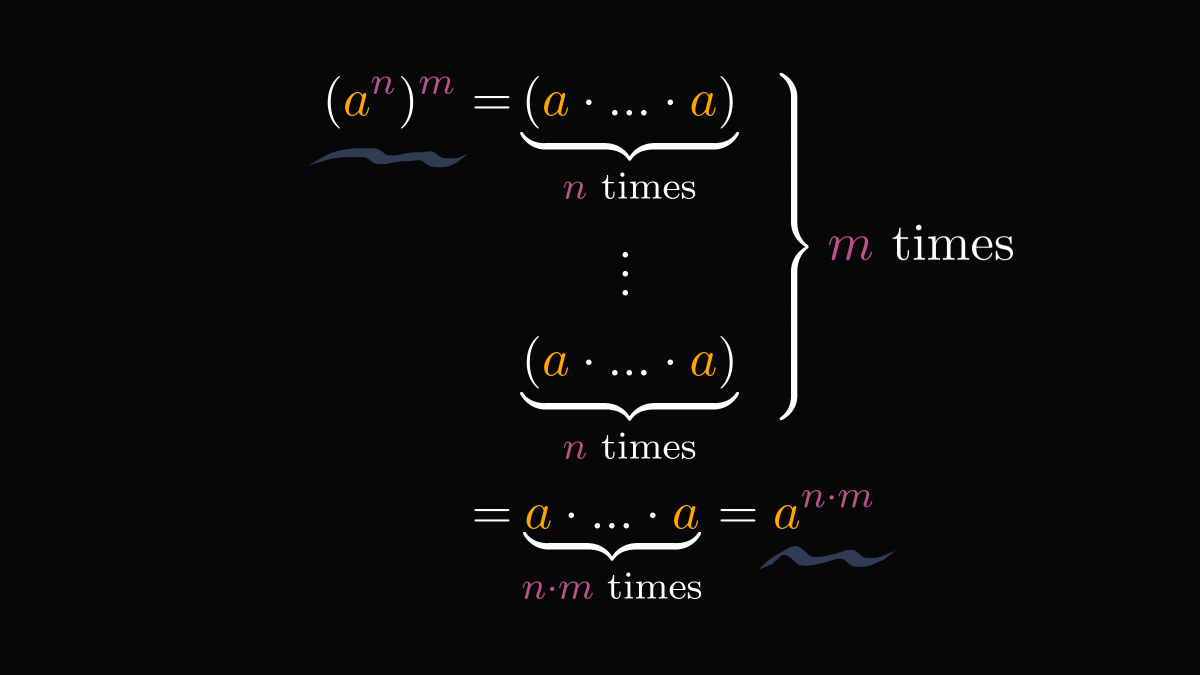We'll call this the "power of powers" rule.

These two identities are the essences of the exponential function. To extend the definition to arbitrary powers, we must ensure that these properties remain true.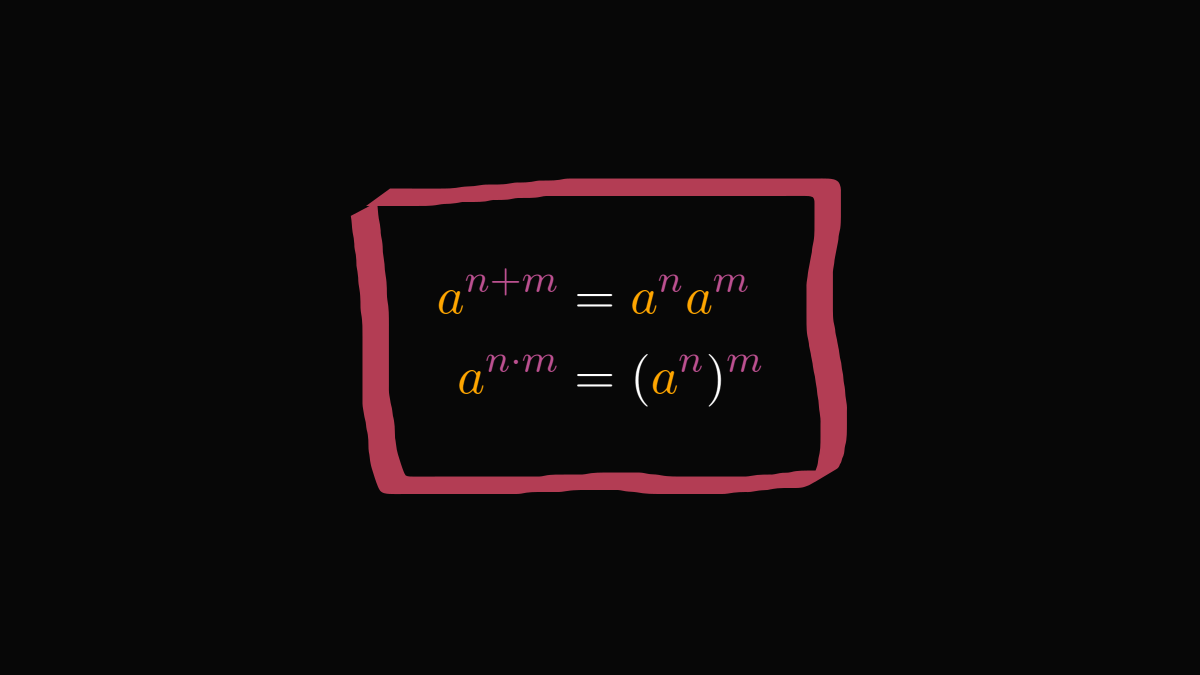So, what about, say, zero exponents? Here, the original interpretation (i.e., repeated multiplication) breaks down immediately. How do you multiply a number with itself zero times?

The "product of powers" property gives the answer. Any number raised to the power of zero should equal to 1.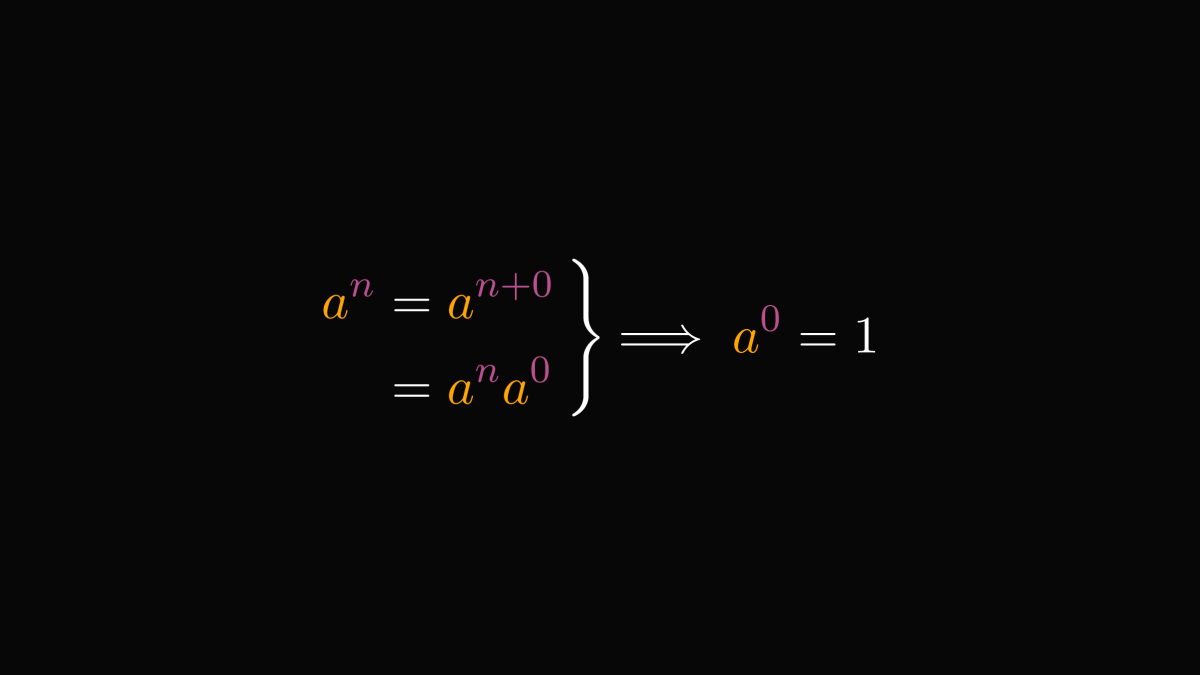What about negative integers? We cannot repeat multiplication zero times, let alone negative times.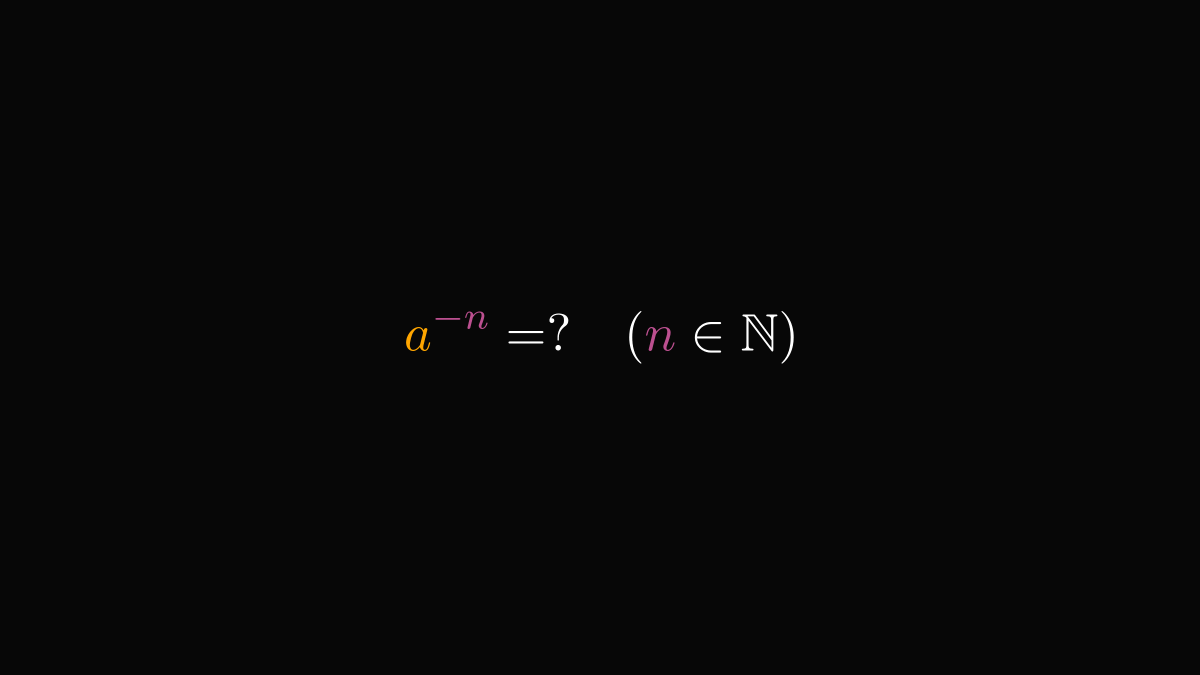Let's use wishful thinking! (I am not kidding. Wishful thinking is a well-known and extremely powerful technique.)

If powers with negative integer exponents are defined, the "product of powers" rule implies that they must satisfy the following.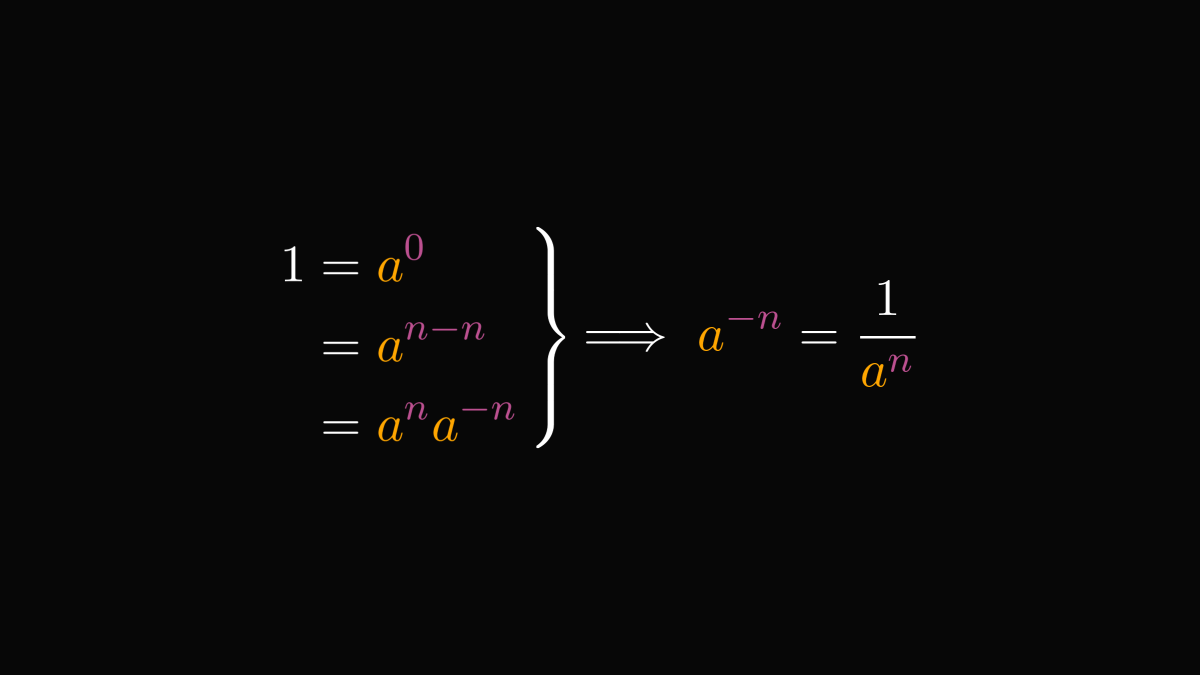So, we can indeed define the exponential for negative integers accordingly.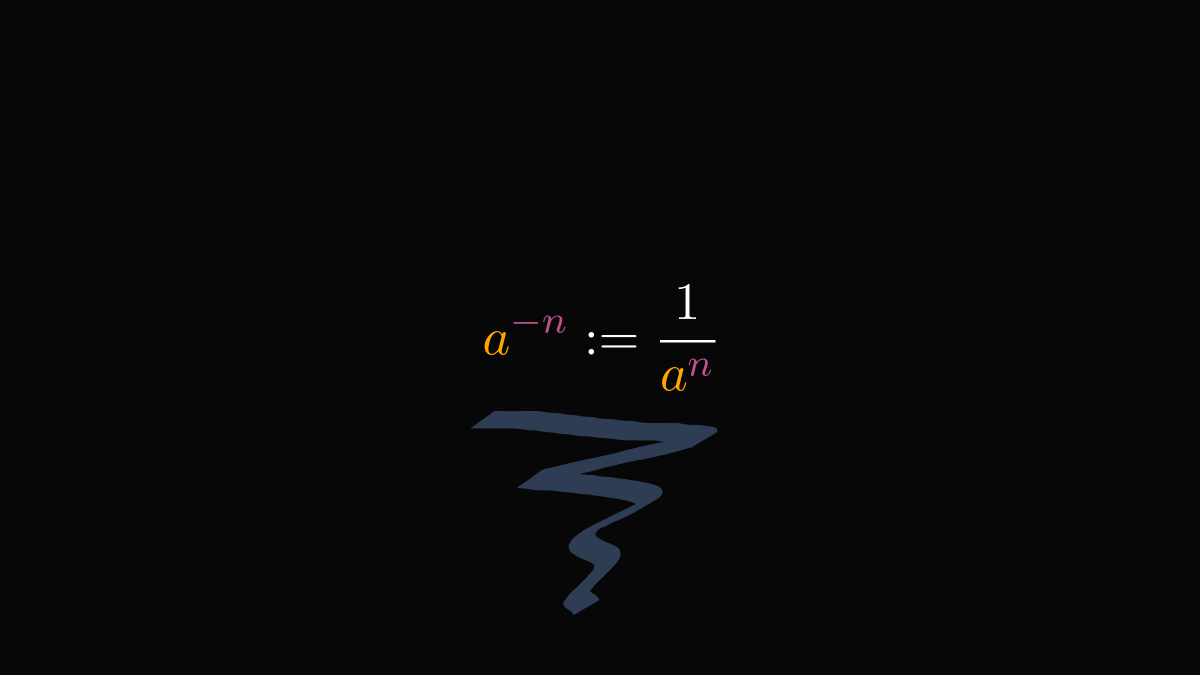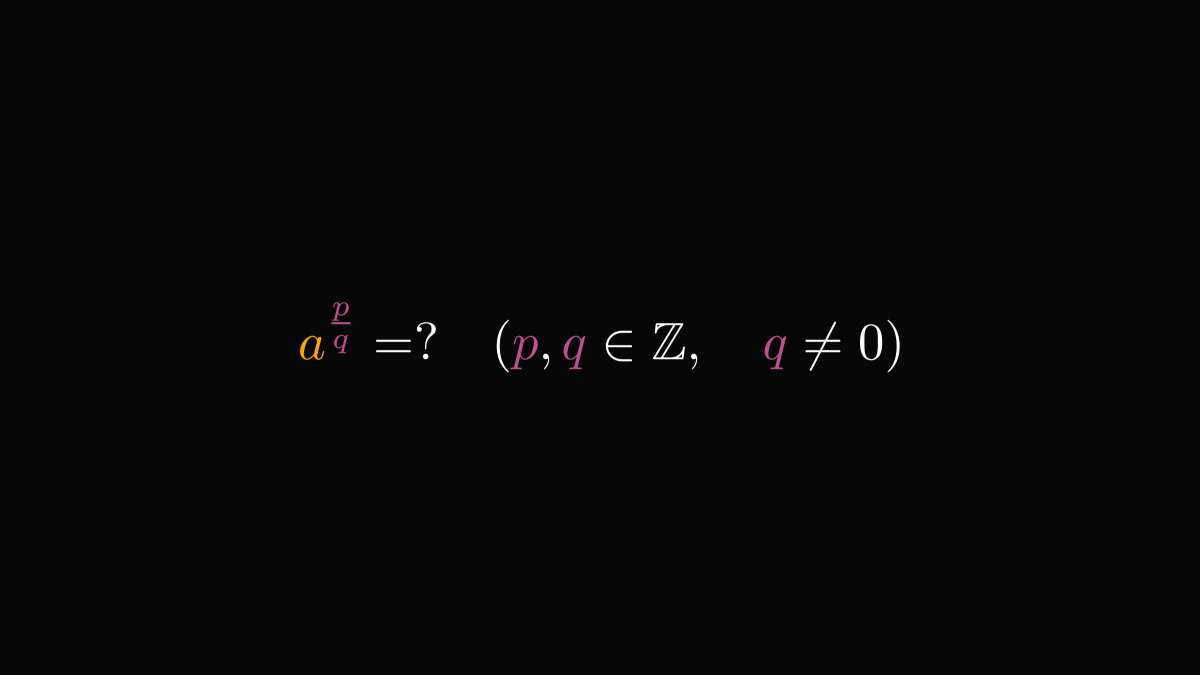You guessed right. Let's use wishful thinking again! The "power of powers" rule yields that it is enough to look at exponents where the numerator is $1$.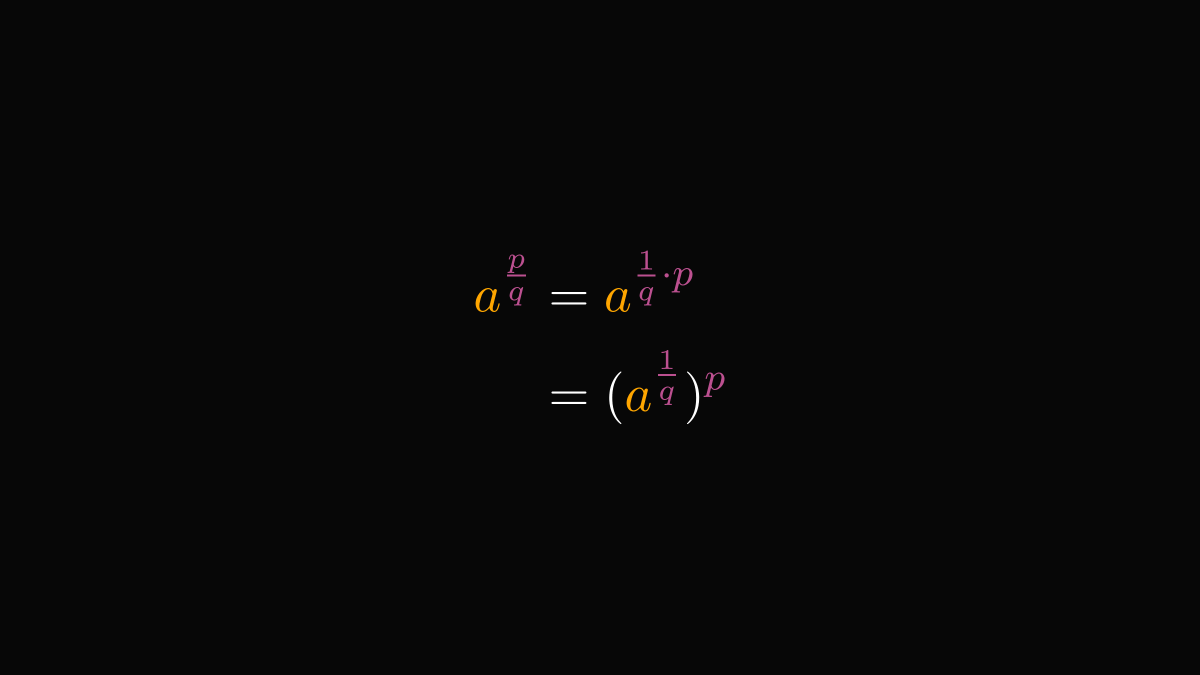The same rule gives that should they exist, they must be defined by the $q$-th root of the base.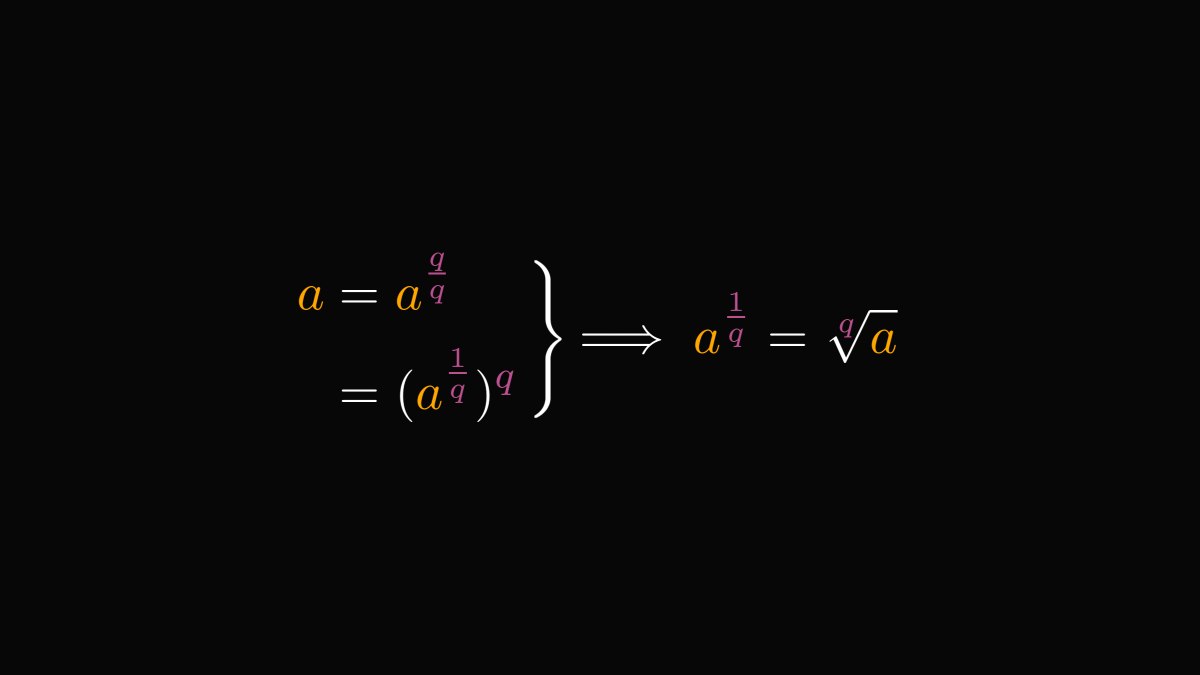Following our usual modus operandi, we use this to define the exponential for rational exponents.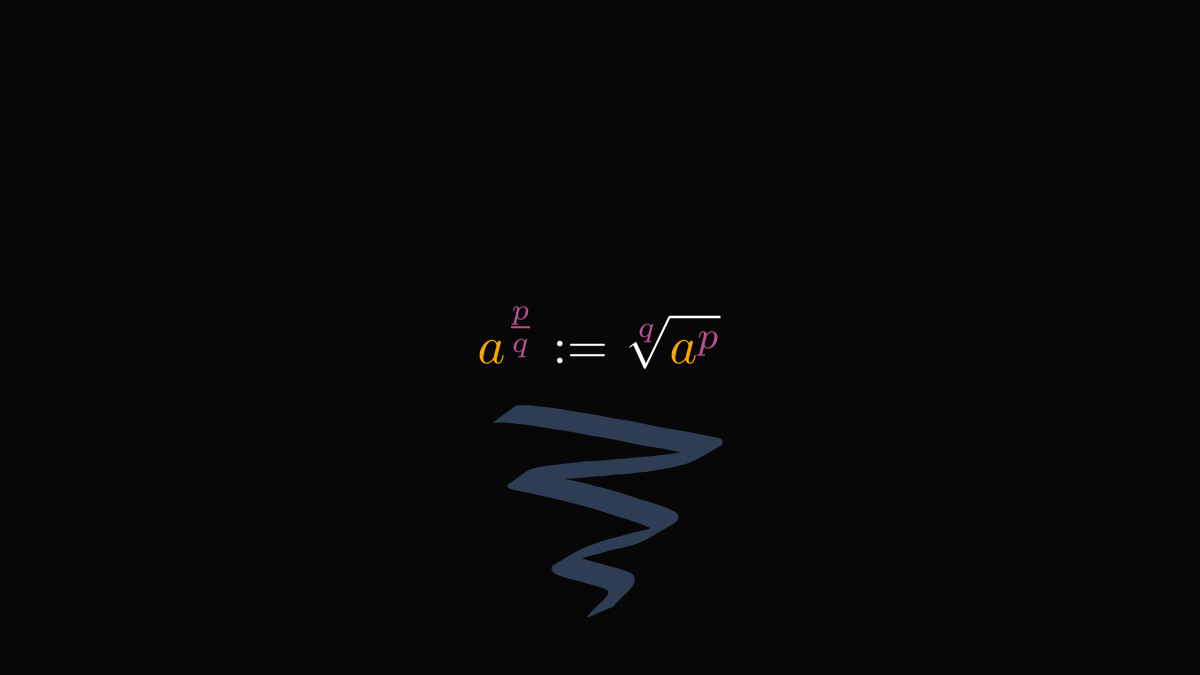Now the big finale: what about arbitrary real numbers as exponents?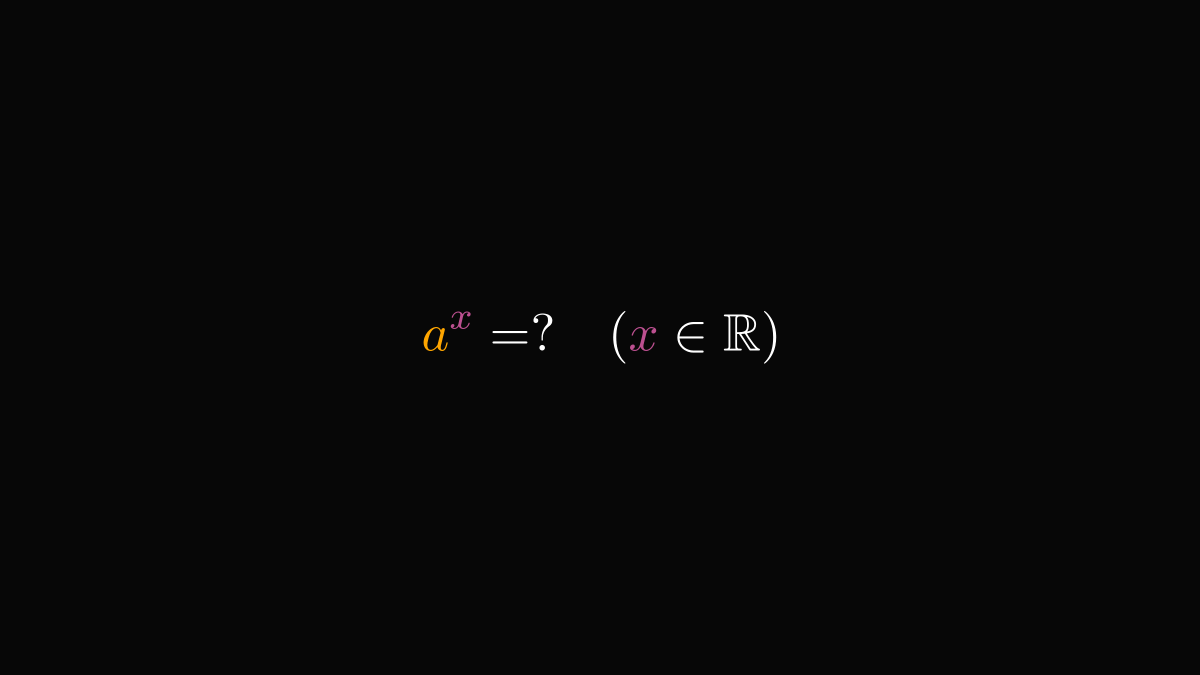Buckle up. We are about to floor the gas pedal. So far, we have defined the exponential function for all rationals. Let's use this to extend it to all real numbers!

Real numbers are weird. Fortunately, they have an exceptionally pleasant property: they can be approximated by rational numbers with arbitrary precision. That is, for all $x \in \mathbb{R}$, there exist a sequence of rationals ${p_n/q_n}_{n=1}^{\infty}$ such that $\lim_{n \to \infty} p_n/q_n = x$.

For instance, this is how π is approximated by rational numbers. Every time you use π inside a computer, an approximation is used. (Most probably not this one.)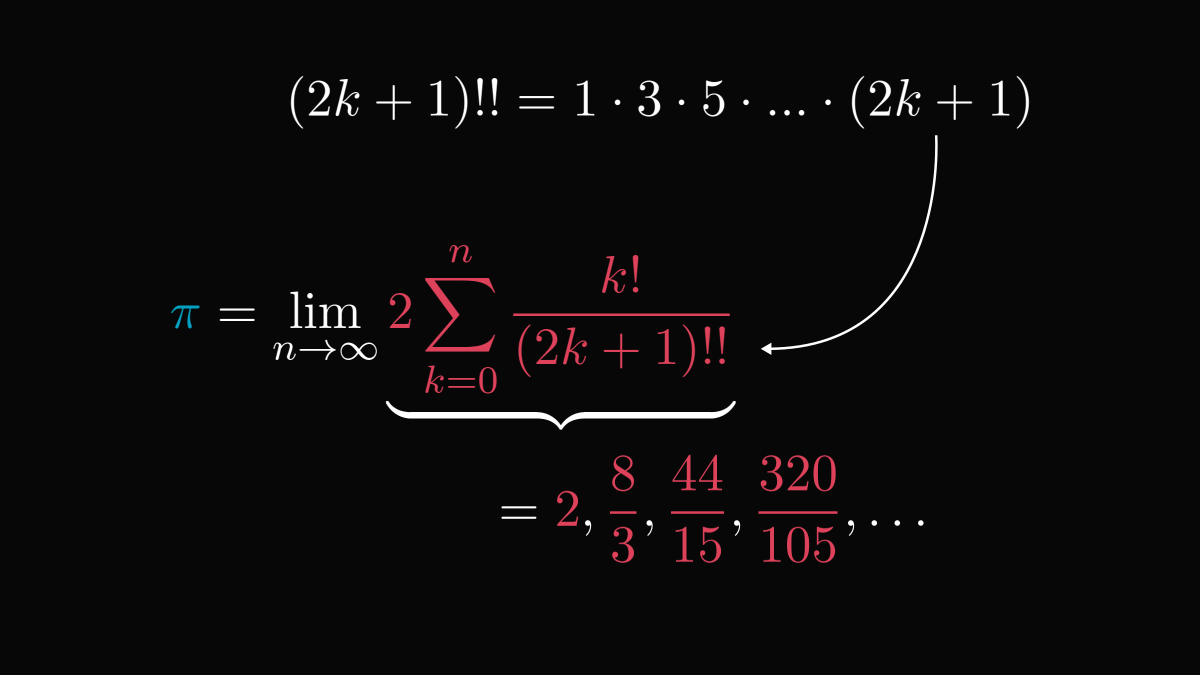When the approximating sequence is close to the actual exponent $x$, the powers are also close. Closer and closer as $n$ grows.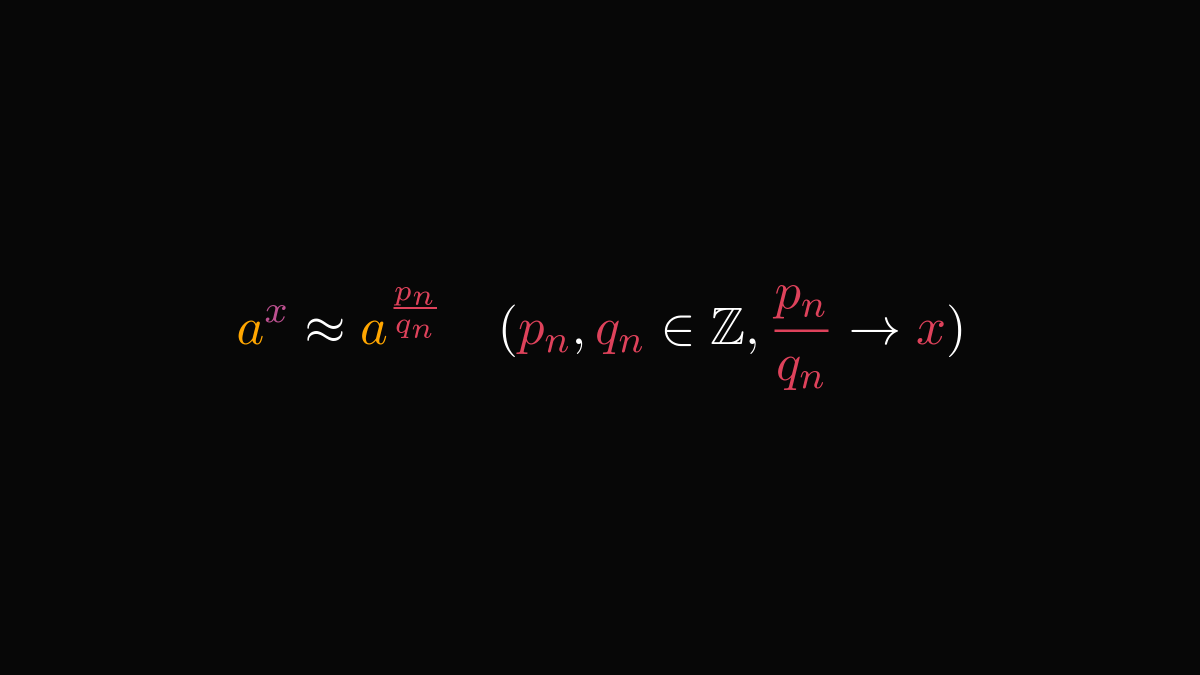Thus, we can define exponentials for arbitrary real exponents by simply taking the limit of the approximations.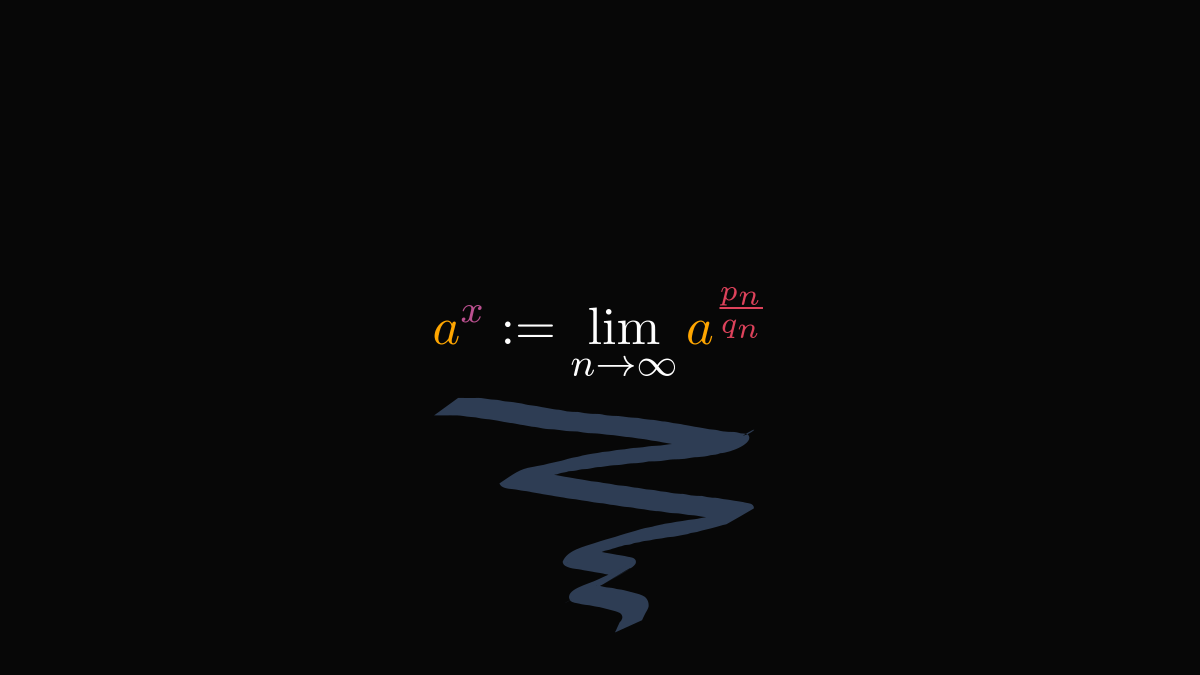You have probably seen the exponential functions thousands of times. We use them every day, yet we hardly ever look behind the surface.